# How to Make a Line Graph in Excel

## Use a Line Chart to Display Data Over Time

Line Graphs are one of the most popular charts used. A line graph has an X axis (horizontal axis on the bottom) and a Y axis (vertical axis to the left). The X axis almost always represents time (hours, days, months, etc.) Therefore, in some industries, a line graph is referred to as a Time Series Chart.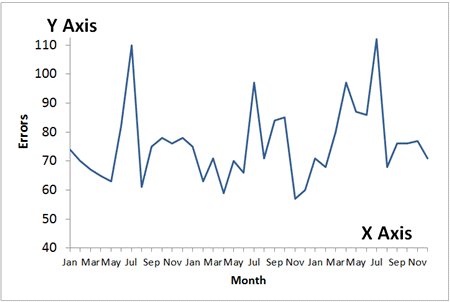### Line Graphs 101

To create a line graph in Excel, select your data, then click on the Insert tab and then Line.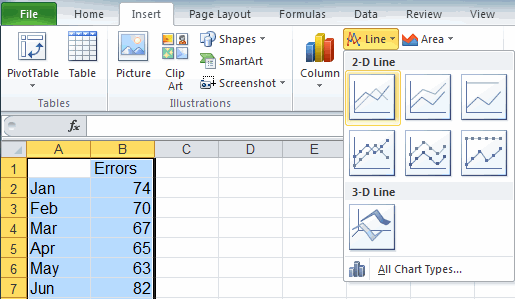### The Evolution of Time Series Charts

While line graphs are a improvement over displaying data in a table or Excel spreadsheet, a run chart or a control chart can actually analyze your data for you.

Line GraphA line graph is the most primitive time series chart.

Run Chart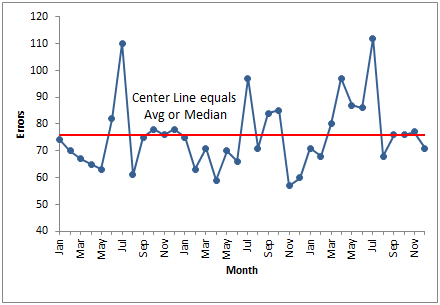A run chart adds a center line which represents the average or the median of the data.

Control ChartA control chart is a smart chart. It calculates a center line and sigma lines. It analyzes the data and identifies unstable points and trends.

Fortunately, control charts are just as easy to create as line graphs using QI Macros Add-in for Excel. See for yourself.

### Improve your data analysis skills using these charts in QI Macros add-in for Excel

Control Charts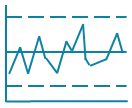Histograms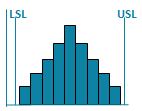Pareto ChartsBox PlotsFishbone Diagram